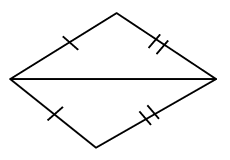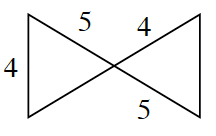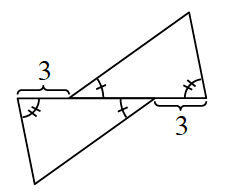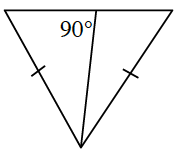### Home > INT1 > Chapter 8 > Lesson 8.1.4 > Problem8-64

8-64.

Identify if each pair of triangles below is congruent or not. Remember that the diagrams may not be drawn to scale. Justify your conclusion. Redraw and label the diagrams as needed.

Read the Math Notes box on triangle congruence conditions.

1.What do the tick marks on the sides mean? Do they help prove the triangles are congruent?

How many sides are congruent in the two triangles is that enough to prove the triangles congruent?

1.How could you prove the triangles are congruent? Is there enough information? Are the parts in the correct order?

1.How do the tick marks on the angles provide enough information to prove they are congruent?

Are there any congruent sides in this figure?

1.What type of triangle is this? What information do you know about the triangle by knowing its name?

What relationship could be used to prove they are congruent?